# Comparing Fractions

Comparing fractions means that you want to be able to tell if the one fraction is less than, greater than, or equal to another.

We can use symbols like we would with whole numbers.

Let's Review:

3 is less than 8 would be written as 3 < 8.

14 is greater than 2 would be written as 14 > 2.

17 is equal to 17 would be written as 17 = 17.

We can do the same thing with fractions.

Let's begin with fractions that common denominators.

1.)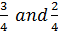When the denominators are the same we can simply compare the numerators.

3 > 2, so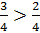2.)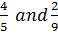When the denominators are not the same, there are several different ways that you can compare.

Method 1:Get common denominators.Now that we have common denominators, we can compare the numerators.

36 > 10, therefore.Furthermore,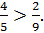3.)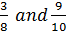Here is another example where the denominators are not the same. Let's look at another method.

Method 2:Use cross products.

When you cross multiply you need to be sure to multiply up! Then write the product at the top of the fraction.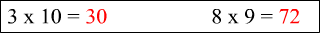Now we will compare the products. 30 < 72 and therefore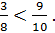4.)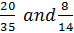Yet another example where the denominators are not the same!

Method 3:Try simplifying the fractions.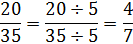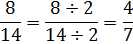Both fraction simplified to the same thing! So we can say that5.)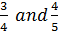Method 4:Change the fractions to decimals.

In this case, you may know the decimal equivalents without much effort.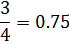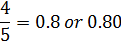Now we can see that 0.75 < 0.80. This means that